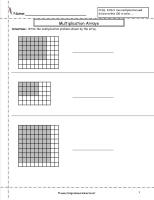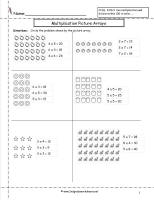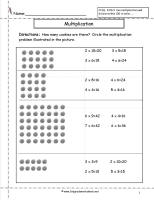﻿ Multiplication Arrays Worksheets

# Multiplication Arrays, Multiplication Arrays Worksheets

## Multiplication Arrays Worksheets, Extension of CCSS 2.OA.4, CCSS 3.OA.3

###Multiplication Arrays Worksheet

Common Core State Standards: Extension of CCSS 2.OA.4, CCSS 3.OA.3
Students write the multiplication problem illustrated by the array.

###Multiplication Arrays Worksheet

Common Core State Standards: Extension of CCSS 2.OA.4, CCSS 3.OA.3
Students illustrate an array to go with the multiplication problem.

###Multiplication Arrays Worksheet

Common Core State Standards: Extension of CCSS 2.OA.4, CCSS 3.OA.3
Students circle the multiplication problem illustrated by the dot array.

###Multiplication Arrays Worksheet

Common Core State Standards: Extension of CCSS 2.OA.4, CCSS 3.OA.3
Students create a dot array to illustrate the multiplication problem.

###Multiplication Arrays Worksheet

Common Core State Standards: Extension of CCSS 2.OA.4, CCSS 3.OA.3
Students circle the multiplication problem illustrated by the picture array.

###Multiplication Arrays Worksheet

Common Core State Standards: Extension of CCSS 2.OA.4, CCSS 3.OA.3
Students circle the multiplication problem illustrated by the cookie array.